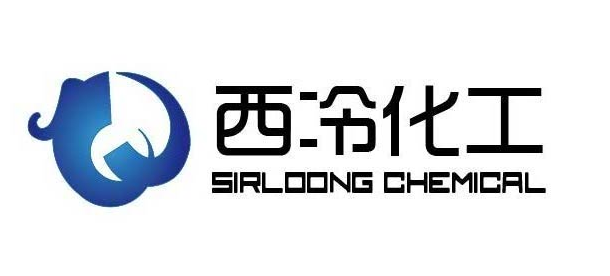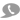15

18653007758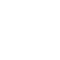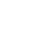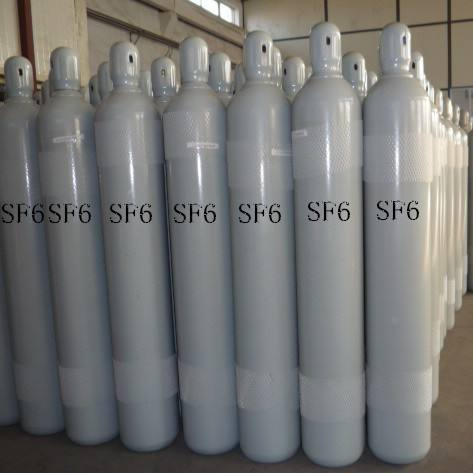1个标准大气压力=1.00336×0.098MPa=0.10108MPa≈0.1Mpa。

1bar=0.1MPa

1MPa=1000kPa， 1kPa=10mbar=101.9716 mmH2O = 4.01463imH2O

10mWC＝1bar＝100kPa，bar 巴 = 0.987 大气压 = 1.02 千克/平方厘米 = 100 千帕 = 14.5 psi

1psi=6.895kPa=0.06895bar

1兆帕 (MPa)=145磅/英寸2(psi)=10.2千克/厘米2(kg/cm2) =10巴(bar)=9.8大气压(atm)

1磅/英寸2(psi)=0.006895兆帕 (MPa)=0.0703千克/厘米2(kg/cm2) =0.0689巴(bar)=0.068大气压(atm)

1巴(bar)=0.1兆帕 (MPa)=14.503磅/英寸2(psi)=1.0197千克/厘米2(kg/cm2) =0.987大气压(atm)

1大气压(atm)=0.101325兆帕 (MPa)=14.696磅/英寸2(psi)=1.0333千克/厘米2(kg/cm2) =1.0133巴(bar)

新闻分类